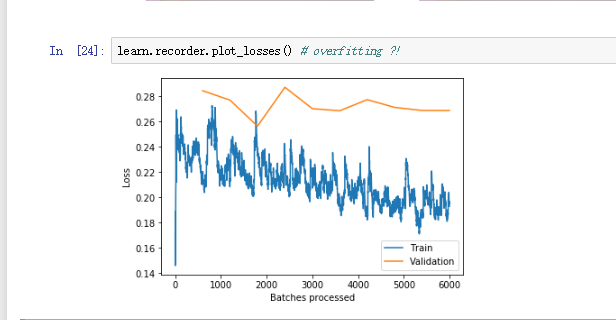# Lesson 3 camvid show_batch() problem

(Karl Mason) #1

I was learning lesson3-camvid.ipynb, all outputs of the previous steps seem correct except when running the following line of code:

`data.show_batch(2, figsize=(10,7), ds_type=DatasetType.Valid)`

the output shows several images, but they all look the same:

no matter how many time i ran the code or change the rows to show.Here is the notebook with strange output that i wrote according to the original notebook, if anywhere was wrong, could you please do me a favor to point it out ?

some of the codes is as follows:

``````%reload_ext autoreload
%matplotlib inline

from fastai.vision import *
from fastai.callbacks.hooks import *
from fastai.utils.mem import *

path = untar_data(URLs.CAMVID)

path_lbl = path/'labels'
path_img = path/'images'

fnames = get_image_files(path_img)
lbl_names = get_image_files(path_lbl)

img_f = fnames
img = open_image(img_f)
get_y_fn = lambda x: path_lbl/f'{x.stem}_P{x.suffix}'

size = src_size // 2
bs = 4
src = (SegmentationItemList.from_folder(path_img)
.split_by_fname_file('../valid.txt')
.label_from_func(get_y_fn, classes=codes))
data = (src.transform(get_transforms(), size=size, tfm_y=True)
.databunch(bs=bs)
.normalize(imagenet_stats))

data.show_batch(2, figsize=(10, 7)) # different outputs every time

data.show_batch(2, figsize=(10, 7), ds_type=DatasetType.Valid)  # outputs always look the same

``````
0 Likes

(Zachary Mueller) #2

If you look at the gentleman on the far right of the screen (he is outlined in black) you can see they are in-fact different photosJust they were taken milliseconds apart! This is due to show_batch() grabbing the first ‘x’ photos in its dataloader. If we assume this is car data continuously being gathered, this behavior would make sense wouldn’t it?

0 Likes

(Karl Mason) #3

Thanks for your quick reply! I used to understand the output as the same way as you said, but it’s more than that. Let me tell you 3 more things:

1. Every time i ran the previous line of code:
``````data.show_batch(2, figsize=(10, 7))
``````

it gave me different images to show. but when i ran

``````data.show_batch(2, figsize=(10, 7), ds_type=DatasetType.Valid)
``````

the output is always look the same…

1. when i ran
``````learn.show_results(rows=3, figsize=(10, 10))
``````

I also got strange result, the first row of output is blank, and the second row is still the same image as above. Also i didn’t get 3 rows of results.

3)Jeremy said in the video that the accuracy(acc_camvid) is hard to reach more than 92.5%, but I got(Strange, isn’t it?). Only it seems that I got overfitting problem?

How can we prevent this or identify a just “right”(not underfit or overfit) result ? Is it the time when train loss is equal to valid loss?0 Likes

(Zachary Mueller) #4

Interesting. I’m wondering if specifying the ds_type resets the tracker for show_batch(). As to the second, I’m not 100% sure as the reasoning for that.

0 Likes

(Karl Mason) #5
0 Likes

(Karl Mason) #6

Maybe the cause of the problem lies in the dataset I downloaded (but I didn’t know why…, I thought everyone downloads the same dataset), delete the dataset and run the notebook from beginning to end again may slove the problem. I rerun the code in colab and it got different results which seems better.

0 Likes